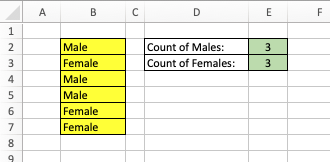Conditional Functions in Excel

Excel has a number of conditional functions available for use in its cell formulas, which are detailed below.

Don't get overwhelmed by all the possibilities here -- the first two functions below $IF()$ and $COUNTIF()$ are the real "workhorses". Know these two functions, and look up the others as special cases arise.

IF (condition, value-if-true, value-if-false)

If the condition evaluates to a TRUE value then the value of the cell containing the IF function is the value of the 2nd argument, while if the condition evaluates to FALSE then the value of the cell containing the IF function is the value of the 3rd argument.

Note that the condition can be built from the logical operators and comparison operators.

For example

$$\begin{array}{ll} =\textrm{IF}(A5 \gt G6, \ldots)\\ =\textrm{IF}(\textrm{AND}(\textrm{NOT}(A7 = B8 + 7), \textrm{SUM}(E2:E7) >= 0), \ldots) \end{array}$$

Know that there are additional special logical functions, such as ISERROR(), ISBLANK(), ISODD(), ISEVEN() and ISNUMBER(), that can help you do certain tests as well.

IF statements can also be nested. E.g. the formula

$$\textrm{IF}(A3 \gt 8, \textrm{"GOOD"},\textrm{IF}(A3 \lt 0,\textrm{"NEG"},\textrm{"BAD"}))$$

returns GOOD if the value in cell A3 is larger than 8, it returns NEG if this value is negative and it returns BAD if A3 has a value between 0 and 8 inclusive.

COUNTIF ($R_1$, criteria)

The value of this function is the number of elements in the range $R_1$ that meets the criteria. Note, for COUNTIF(), $R_1$ can be any $m \times n$ range.

When the values in array $R_1$ are alphanumeric, the criteria takes forms such as "Male" or B5. Consider the following example:E2 : "=COUNTIF(B2:B7,"Male")"
E3 : "=COUNTIF(B2:B7,"Female")"

Wildcards can also be used in the criteria; e.g. "*ite" selects all values in $R_1$ which end in the letters "ite". Selection is case-insensitive, i.e. "Male" is equivalent to "MALE" or "mALe".

You can also use criteria such as ">="&"M" to select all values in $R_1$ which start with the letter M or higher.

Some additional examples of criteria and ways to use COUNTIF are given below:

COUNTIF(R,">"&B3*2)
yields the number of cells in range R that are bigger than twice the value in cell B3 (note, the function of the ampersand, &, above is to concatenate the string ">" with the string of text associated with the value of B3*2)

COUNTIF(R,10)+COUNTIF(R,-10)
yields the number of cells in range R equal to 10 or -10

COUNTIF(R,"???")
yields the number of cells in R that contain exactly 3 characters

COUNTIFS ($R_1$, criteria1, $R_2$, criteria2, ...)

This function is identical to COUNTIF(), except that one can provide multiple criteria. As an example of when one might use this function, consider when one wishes to count the values some range $R$ that are in an interval $(a,b)$. That is to say, each value $x$ in the range $R$ should only be counted if $x > a$ and $x < b$. One criteria could take the form ">a", while another could take the form "<b".

IFS (logical-test1, value-if-test1-true, logical-test2, value-if-test2-true, $\ldots$)

As described above, you can nest IF statements. The problem with this is that the nesting (and especially the placement of parentheses) can become complicated. Excel 2016 introduces a new IFS function which simplifies such formulas. For example, the following are equivalent: $$\textrm{IF}(A3 \gt 8, \textrm{"GOOD"},\textrm{IF}(A3 \lt 0,\textrm{"NEG"},\textrm{"BAD"}))$$ $$\textrm{IFS}(A3 \gt 8, \textrm{"GOOD"}, A3 \lt 0, \textrm{"NEG"},\textrm{TRUE},\textrm{"BAD"})$$

SWITCH (expression, value1, result1, value2, result2, $\ldots$)

Excel 2016 also provides a new conditional function similar to IFS, which returns the first resultN where expression = valueN.

SUMIF ($R_1$, criteria, $R_2$)

$R_2$ is an array of potential values to be summed and $R_1$ is an array of the same shape and size containing values to be matched against the criteria. For each value in $R_1$ that meets the criteria, the corresponding value in $R_2$ is used in the sum. Where the values in $R_1$ are numeric, criteria can take the form of a constant such as 34 or B5, or a logical expression (in quotes) of form ">34", "<>34", "<=34"or ">"&B5.

Note that you need to use the ampersand (i.e. the concatenation operator) when creating logical expressions that refer to a cell's value. Thus the "<="&B5 criteria is met for all cells in R1 which are less than or equal to the value in cell B5.